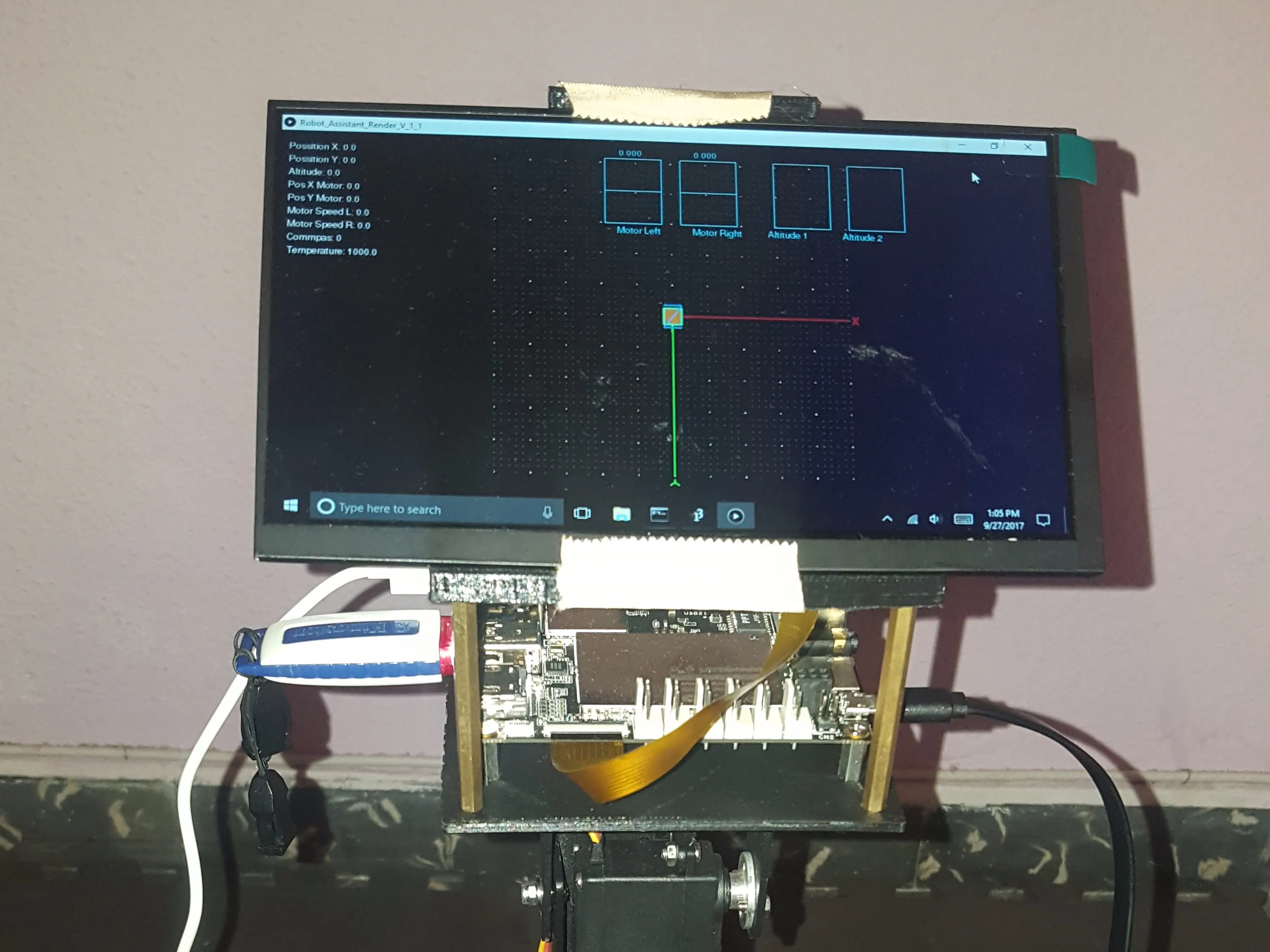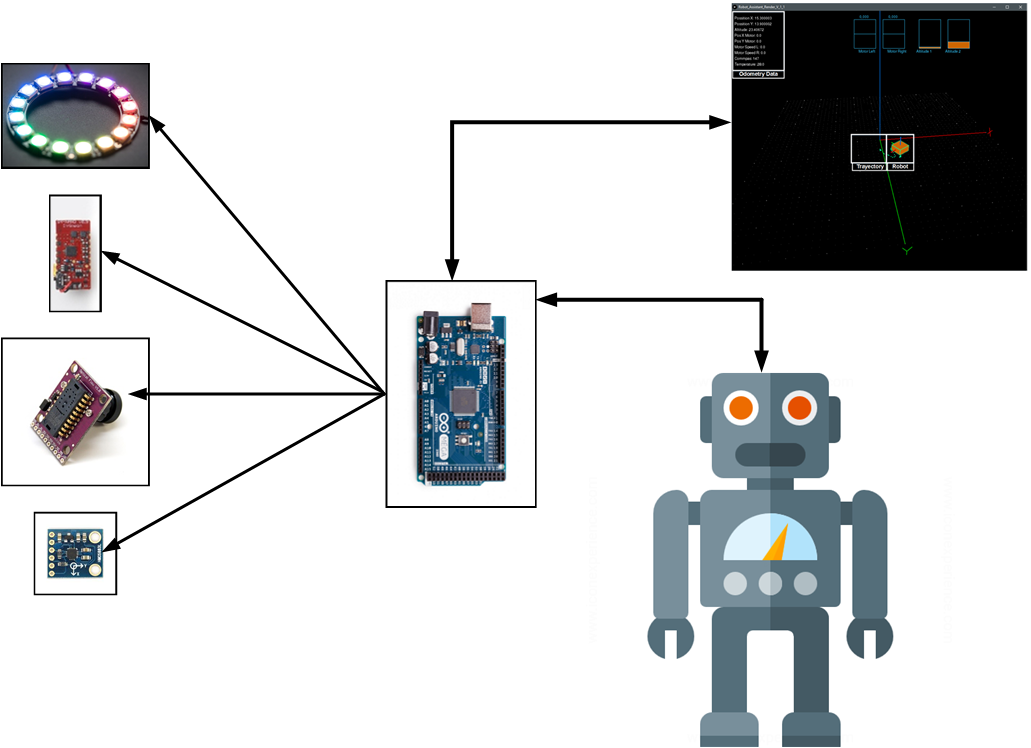The new sensors of measurement of atmospheric pressure allow to calculate the height of a space having as reference the level of the sea.

• 251 views
• 2 respects

## Necessary tools and machinesSoldering iron (generic)

## Apps and online services

Introduction

In the field of mobile robotics, determining the position of a robot within a closed environment remains a topic of debate. Currently, to determine the positioning of an object in a closed environment, several techniques are used such as SLAM, 3D mapping, pattern recognition, among others. These positioning tools allow robots to navigate within a home or office. However, it is still difficult to determine precisely where in the closed environment the robot is located, for example in a living room or in the kitchen of a house. For this, a series of QR markers are used which indicate to the robot its exact position. These identification processes allow the robot to determine its position through image processing techniques.

The new sensors of measurement of atmospheric pressure allow to calculate the height of a space having as reference the level of the sea. Therefore, these sensors could help the robots stablish where in the closed environment they are, taking into account that some places of an enclosure like a house can be in a floor or in another higher one.

Objetive

This project has as main objective to determine the exact position of a robot in a closed environment, taking into account the variations of height. For this purpose, the following sub-objectives were proposed.

• Build a mobile robotic platform.

• Characterize the sensor.

• Program the scheduling algorithm using AI.

Due to the complexity of the project, only the first two sub-objectives were completed.

Hardware

For the construction of the aluminum structure of the robot has been used makerbeam (Figure 1)

A Nano Sensor Hub development card which is built with a DPS310 sensor (Figure 2).

This sensor has the following technical characteristics:

• Operation range: Pressure: 300 –1200 hPa.

• Temperature: -40 – 85 °C.

• Pressure sensor precision: ± 0.005 hPa (or ±0.05 m) (high precision mode).

• Relative accuracy: ± 0.06 hPa (or ±0.5 m) • Absolute accuracy: ± 1 hPa (or ±8 m)

• Temperature accuracy: ± 0.5°C.

• Pressure temperature sensitivity: 0.5Pa/K

The robot has an ADNS 3080 optical flow sensor (Figure 3) which measures the amount of movement or displacement of a two-dimensional plane by image processing techniques.

In addition, a ring of RGB leds (Figure 4) was arranged, which can modify the color of the led depending on the surface, in order to improve image acquisition through the ADNS3080 sensor and to normalize the color of the surface to which the sensor points to.

A digital magnetometer hmc5883l (Figure 5) designed for low field magnetic detection with a digital interface for applications such as low cost compass and magnetometry.

The robot control process was divided into two stages. A low-level stage using a Mega Arduino 2560 (Figure 6), which is responsible for motor control, communication with the DPS310 and another series of sensor that help the robot to determine its position.

The second control stage is performed using a Lattepanda (Figure 6) with its respective LCD. This stage is responsible for the calculation of height, position and sending control data for the robot (distance, angle of rotation and color of the ring of leds).

Software Description

In order to graph the movements of the robot as it moves within the environment a program was developed using processing. This program is a 3D visualization environment in which the robot is represented as a cube and it is possible to observe the trajectory traversed by the robot and changes in altitude as the robot moves (Figure 7).

The information sent by the mega arduino to the graphical interface has been coded as a list. This list contains the sensor information, as shown in Figure 8.

The message starts with the character "@" followed by the position in (x, y) acquired by the sensor ADNS3080. The parameter "surfaceQuality" indicates the quality of the surface on which the robot is located. v_M1 and v_M2 are the velocities of the robot and x_Motor and y_Motor represents the position in (x, y) calculated using the rotations of the motors. The pressure parameter and temperature are obtained through the DPS310. Encode_M2 and Encoder_M1 are the tics of the encoder and these increase as the robot advances. Angle_X, Angle_Y, Angle_Z are the angles acquired through the IMU and finally the compass variable witch is messured using the hmc5883l sensor. This values are used to know the angle regarding the magnetic north of the earth.

To calculate the engine speed the position of the motors in (x, y) the following formulas were used.

X=Distance*Cos(Theta)

Y= Distance*Sin(Theta)

Equation 1: Calculating the position using the motore

Where distance is the distance traveled by the robot. This parameter depends on the diameter of the wheels which in the case of this robot is 5cm.Theta is the angle of rotation acquired by the compass.

The calculation of the height using barometric pressure and temperature is described in Equation 2.

H = (((sea_press/press)^ 1 / 5.257) – 1.0) * (temp + 273.15)) / 0.0065

Equation 2: Calculation of Height

Where "sea_press" is the barometric pressure where the robot is in relation to the level of the sea. "Press" is the pressure sent by the sensor and "temp" is the temperature of the place where the robot is located. This last calculation is performed in lattepanda and is displayed on two slides (Figure 8).

The first slide (Altitude 1) shows the height in meters and the second slide (Altitude 2) shows the height in centimeters.

The next graph show somo experimente doing with the robot, the first graph show a plane surface and the second graph show a surface with a some high change.

Robot:

Conclusion:

This project presents a new way to use a pressure sensor into a robot. This new way allows to the robot acquire height variations in our homes. This variation could be used to planified the movement of the robot into complex environment. to made this planfication is necesary use different algorithm of artificial intelligent, to recognice the robot position. At the same time, we present a grafical user interface (GUI) that shows all the information sending by the low level control. This GUI shows the altidude and the robot position.

## Custom parts and enclosures

designing with solidworks
infineon_hub_nano_base_L3URo2yDAv.SLDPRT
base_latte_panda_zse7IKzPQ8.SLDPRT
base_latte_panda_1_rioI4KxzQM.SLDPRT

## Schematics

Since the robot has a high complexity, I decided to make a block diagram.